#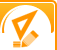Math and Gender## Purpose

To determine if there is a difference between the overall math test scores of girls and boys and to determine if this is a direct reflection of gender related superior mathematical ability.

Many scientific sources have speculated that boys are better than girls at math. While statistical data may suggest that boys are in fact, a little better at solving mathematical problems than their female counterparts, you can conduct a simple experiment in your own school to prove or disprove this theory.

## Required materials

• A short math quiz with grade appropriate problems (developed by you or a math teacher) with an answer key
• Pencils
• Calculators (if required) or you can have students use their own
• Calculator for your end of experiment calculations
• Timer
• Several student volunteers with an equal mix between boys and girls
• Notebook paper or logbook

## Estimated Experiment Time

This experiment is likely to take a few hours.

## Step-By-Step Procedure

• 1. Have the student volunteers assemble in the cafeteria at tables or at desks in a classroom if a teacher will allow you to do so for your experiment.
• 2. Pass out the tests (face down) to each student along with a pencil. You can also pass out calculators at this time or ask students to retrieve their own.
• 3. Set the timer for 15 minutes. The test should be short enough that the students can complete the test in this amount of time or less.
• 4. Start the timer and allow students to begin the test. Have each student write down their gender on the top of the page, but not their name.
• 5. When the students have completed the test, have them turn the tests in to you. You will then proceed to grade the tests.
• 6. Once the tests are graded, calculate what percentage of girls got 100% of the answers correct on the test and what percentage of boys got 100%. Do this for each corresponding percentage bracket, such as 90%, 80%, etc.

## Note

You will most likely need a fair number of student volunteers so you can gather enough statistical data for an accurate conclusion.

## Observation

It is important that you calculate everything correctly, so be sure and double check your answers and calculations for consistency and accuracy.

## Result

The result all depends on what your statistical data supports. Did the boys in your experiment get better math test scores than the girls or was it the other way around? Did the boys and girls in your experiment test equally? What do you think is responsible for the difference in scores, if there is one? After the experiment, what is your stance on the theory that boys are better at math than girls?

Take a moment to visit our table of Periodic Elements page where you can get an in-depth view of all the elements, complete with the industry first side-by-side element comparisons!

 Message: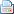Print this page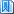Bookmark this pageHide all projects# All Projects List

•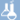Chemistry
•Biology
•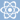Physics
•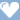Medicine & Health
•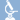Biochemistry
•Environmental science
•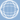Earth science
•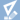Mathematics
•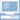Computer science